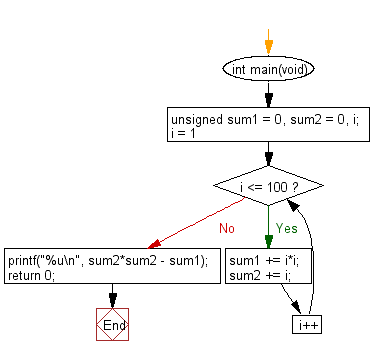﻿ C Program: Squared sum minus square of 1st 100 numbers# C Exercises: Find the difference between the sum of the squares of the first one hundred natural numbers and the square of the sum

## C Programming Practice: Exercise-22 with Solution

The sum of the squares of the first ten natural numbers is,
12 + 22 + ... + 102 = 385
The square of the sum of the first ten natural numbers is,
(1 + 2 + ... + 10)2 = 552 = 3025
Hence the difference between the sum of the squares of the first ten natural numbers and the square of the sum is 3025 − 385 = 2640.
Write a C programming to find the difference between the sum of the squares of the first one hundred natural numbers and the square of the sum.

C Code:

``````#include <stdio.h>
int main(void)
{
unsigned sum1 = 0, sum2 = 0, i;
for (i = 1; i <= 100; i++) {
sum1 += i*i;
sum2 += i;
}
printf("%u\n", sum2*sum2 - sum1);
return 0;
}
``````

Sample Output:

```25164150
```

Flowchart:## C Programming Code Editor:

What is the difficulty level of this exercise?

Test your Programming skills with w3resource's quiz.

﻿

## C Programming: Tips of the Day

Maximum value of int:

In C:

```#include <limits.h>
then use
int imin = INT_MIN; // minimum value
int imax = INT_MAX;```

or

```#include <float.h>

float fmin = FLT_MIN;  // minimum positive value
double dmin = DBL_MIN; // minimum positive value

float fmax = FLT_MAX;
double dmax = DBL_MAX;```

Ref : https://bit.ly/3fi8yk9